# Electrical Conductor: What is it? (Diagram & Types of Conductors)

Contents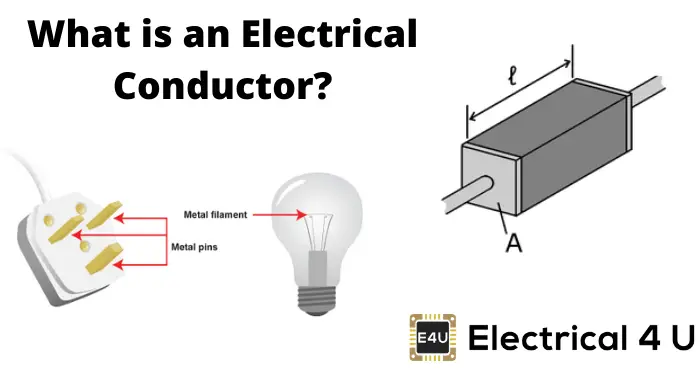## What is an Electrical Conductor?

In electrical engineering, a conductor (or electrical conductor) is defined as an object or type of material that allows the flow of charge in one or more directions. Materials made of metal are common electrical conductors, as metals have a high conductance and low resistance.

Electrical conductors allow electrons to flow between the atoms of that material with drift velocity in the conduction band. Electrical conductors may be metals, metal alloy, electrolyte, or some non-metals like graphite and a conductive polymer. These materials allow electricity (i.e. the flow of charge) to pass through them easily.

## How Does a Conductor Conduct Current?

The substance of the electrical conductor atom must have no energy gap between its valence band and conduction band.

The outer electrons in the valence band are loosely attached to the atom. When an electron gets excited due to electromotive force or thermal effect, it moves from its valence band to the conduction band.

The conduction band is the band where this electron gets its freedom to move anywhere in the conductor. The conductor is formed of atoms. Thus as a whole, the conduction band is in the abundance of electrons.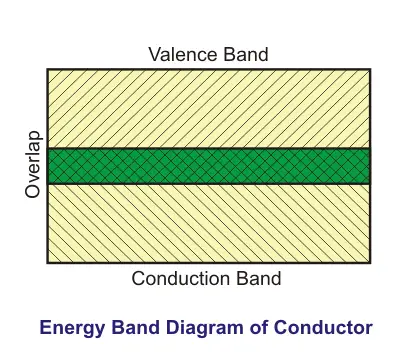In other words, it can be said that the metallic bonds are present in the conductors. These metallic bonds are based on the structure of positive metal ions. These structures are surrounded by an electron cloud.

When a potential difference occurs in the conductor across two points, the electrons get sufficient energy to flow from lower potency to higher potency in this conduction band against a small resistance offered by this conductor material. Electricity or current flows in the opposite direction of the flow of the electrons.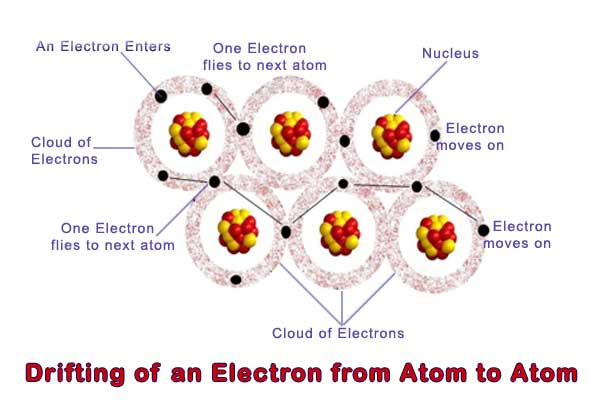## How an Electron Flows through a Conductor?

Electrons do not move or flow in a straight line. In a conductor, the electrons are in to and fro motion or random velocity i.e. is called Drift Velocity (Vd) or average velocity. Due to this Drift Velocity, the electrons get collisions every moment with atoms or another electron in the conduction band of the conductor.

Drift velocity is quite small, as there are so many free electrons. We can estimate the density of free electrons in a conductor, thus we can calculate the drift velocity for a given current. The larger the density, the lower the velocity required for a given current.

In the Conductor, the flow of the electrons is against the Electric Field (E).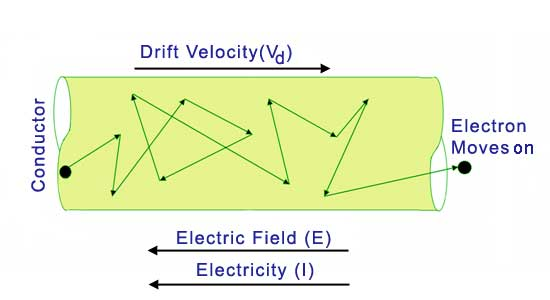## Properties of Electrical Conductor

The main properties of electrical conductors are as follows:

1. A conductor always allows the free movement of electrons or ions.
2. The electric field inside a conductor must be zero to permit the electrons or ions to move through the conductor.
3. Charge density inside a conductor is zero i.e. the positive and negative charges cancel inside a conductor.
4. As no charge inside the conductor, only free charges can exist only on the surface of a conductor.
5. The electric field is perpendicular to the surface of that conductor.

## Type of Conductors

Electrical conductors can be classified based on their Ohmic Response. They are:

### Ohmic Conductors

This type of conductors always follow Ohm’s Law (V ∝ I)
V vs. I graph gives a straight line always.
Example
Aluminum, Silver, Copper etc.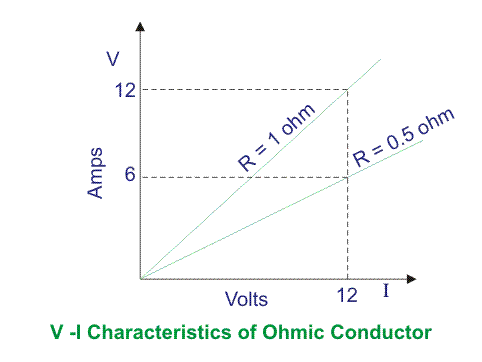### Non-Ohmic Conductors

This type of conductors never follow Ohm’s Law (V ∝ I)
V vs. I graph does not give a straight line i.e. nonlinear graph.
Example
LDR (Light Dependent Resistor), Diode, Filament of Bulb, Thermistors, etc.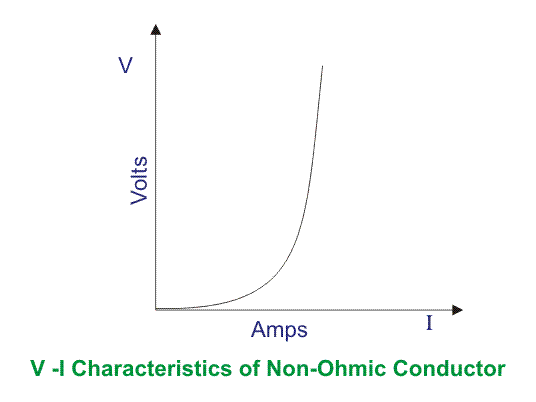The examples of conductors are given below

### Solid Conductor

1. Metallic Conductor: Silver, Copper, Aluminum, Gold, etc.
2. Non Metallic Conductor: Graphite
3. Alloy Conductor: Brass, Bronze, etc.

### Liquid Conductor

1. Metallic Conductor: Mercury
2. Non Metallic Conductor: Saline Water, Acid Solution, etc.

NB:

1. Copper Conductor is the most common material used for electrical wiring.
2. Gold Conductor is used for high-quality surface-to-surface contacts.
3. Silver is the best conductor on the Conductors list.
4. Impure Water is listed in the Conductor List but it has less conductivity.

## What is the Charge of a Conductor During Carrying Electricity?

A current-carrying conductor at any instance has zero charge. This is because at any instance number of electrons (at drift velocity) is equal to the number of protons in this conductor. So the net charge is zero.

Suppose a conductor is connected across a battery, i.e. positive end and negative end are connected with a conductor. Now electrons flow through the conductor from the negative end to the positive end of the battery. This flow of electrons is possible until this battery has EMF producing capability through a chemical reaction inside.

## Is A Conductor Positively or Negatively Charged?

Just think that here the conductor is the media through which charges can be passed from one electrode to another electrode of the battery. The electrons get rid of the negative side of the battery and enter the conduction band of the conductor where already plenty of valence electrons of conductor atoms are available.

The free electrons start the journey in drift motion (towards the positive electrode of the battery) from atom to atom in the conduction band.

At any instance, each atom holds zero charge because drift electrons from adjacent atoms fill its valence band electron gaps and it happens continuously i.e. total number of electrons equal to the number of protons in the conductor at any moment. Now the rate of change of charge (q) with respect to time (t) is called current (I),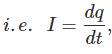This rate of change of charge with respect to time occurs. Convention-wise, Current (I) flows in the opposite direction of electron flow.

When you remove the conductor from the battery, this conductor does not hold any charged particle, but EMF remains present across the battery electrodes with positive and negative polarity with no flow of electrons.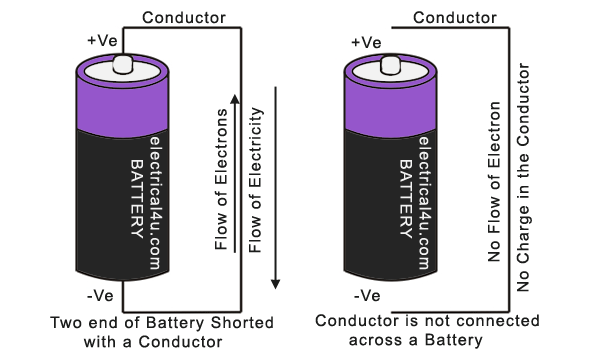## Effect of Temperature on a Conductor

The more effect of temperature, the more vibration in the conductor molecules. This impedes the electrons to flow, i.e. the electrons get obstruction to flow smoothly through the conductor. Thus conductivity decreases gradually with increasing the temperature.

Again, raise of temperature break some bonds in the conductor molecules and release some electrons. These electrons are less in number. As a whole, it can be said that increase in the temperature opposition against the drifting electron increases in the conductor.

Want To Learn Faster? 🎓
Get electrical articles delivered to your inbox every week.
No credit card required—it’s 100% free.